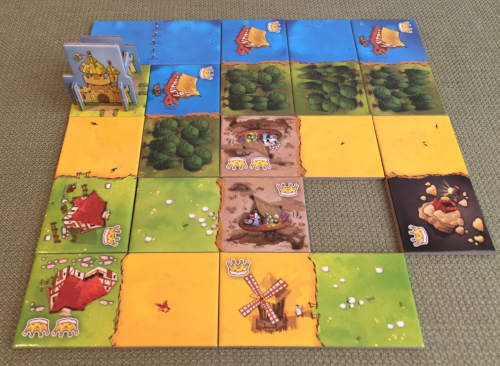# Kingdomino

A multiplayer game in which colored dominoes are tiled in a square square board to maximize a weighted sum of the monochromatic regions' sizes.## Description

Kingdomino is a multiplayer game played with a multiset $S$ of colored dominoes, whose total number $\mid S \mid$ is a multiple of the number of players $p$. Each of the two $1 \times 2$ cells $c$ of a domino $d \in S$ is colored with one out of $k$ colors and is associated with a non-negative integer $\ell(c)$.

Each player has a personal grid that will host a kingdom consisting of non-overlapping polyominoes. The cell at coordinates $(0, 0)$ is occupied by a special castle monomino and there is a fixed upper bound $h$ on the size of the kingdom (as explained in the following).

Initially, the dominoes in $S$ are shuffled into a random sequence unknown to the players. Then, the game proceeds in $|S|/p$ phases. In each phase a batch containing the next $p$ dominoes of the sequence is revealed, and each of the players selects a distinct polyomino from the batch (from the second batch onward, the $i$-th player to choose is the one that selected the $i$-th domino in the previous batch).

Each player then has to place the newly selected domino $d$ into it their grid such that:

• $d$ does not overlap any other polyomino; and
• the non-empty cells of the resulting grid fit into a $h \times h$ subgrid.

Additionally, at least one of the following two conditions must be satisfied:

• A cell of $d$ is adjacent to $(0,0)$; or
• A cell of $d$ shares an edge with a nearby cell of an already-placed domino, and both cells have the same color.

If no such valid placement exists, then $d$ is discarded.

At the end of the game, each player computes their score by considering the monochromatic connected components $C_1, C_2 \dots$ (w.r.t. $4$-adjacency) induced by the non-empty cells in the player’s grid. The final score of the player is then $\sum_i |C_i| \cdot \left( \sum_{c \in C_i} \ell(c) \right)$, where $|C_i|$ denotes the number of cells in $C_i$.

The player with the highest score wins the game.

In the classical version of the game (shown in the picture) $k=h=5$, and $\ell(c)$ is shown by the number of crowns in the corresponding cell $c$. The score of the shown kingdom is (from top to bottom, from left to right) $6 \cdot 3 + 3 \cdot 0 + 1 \cdot 0 + 1 \cdot 0 + 2 \cdot 3 + 2 \cdot 0 + 3 \cdot 3 + 1 \cdot 1 + 2 \cdot 1 + 1 \cdot 0 = 36$.

## Computational complexity

The version of the game in which the players know in advance the sequence of dominoes that they will have to place has been studied in . For this version, the problem of deciding whether a fixed player can score at least a given number of point is NP-complete .

 V.-H. Nguyen, K. Perrot, M. Vallet, “NP-completeness of the game Kingdomino™”, Theoretical Computer Science, 2020.

Image courtesy of www.TheBoardGameFamily.com.

@misc{cog:kingdomino,
author = "{CoG contributors}",
title  = "{Kingdomino --- Complexity of Games}",
year   = "2022",
url    = "https://www.isnphard.com/i/kingdomino/",
note   = "[Online; accessed 2022-06-02]"
}